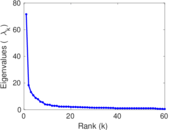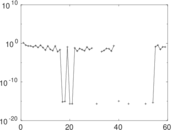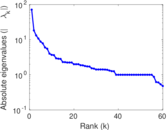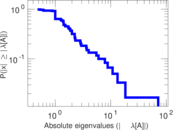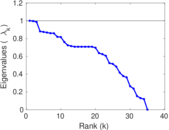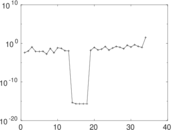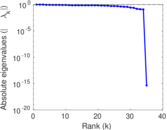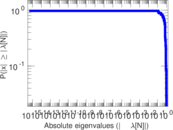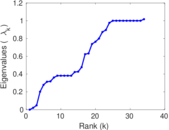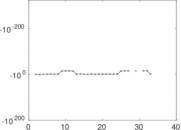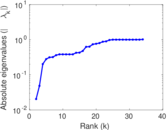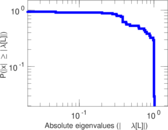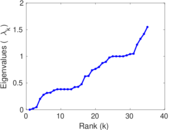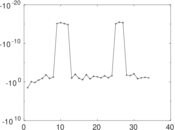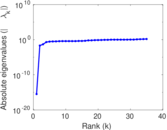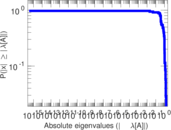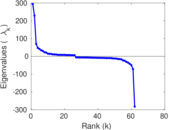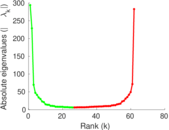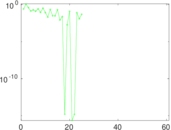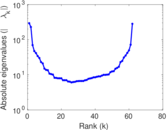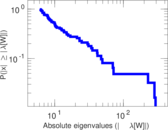Wikipedia edits (ii)

This is the bipartite edit network of the Sichuan Yi Wikipedia. It contains users and pages from the Sichuan Yi Wikipedia, connected by edit events. Each edge represents an edit. The dataset includes the timestamp of each edit.

 Code ii Internal name edit-iiwiki Name Wikipedia edits (ii) Data source http://dumps.wikimedia.org/ AvailabilityDataset is available for download Consistency checkDataset passed all tests Category Authorship network Dataset timestamp 2017-10-20 Node meaning User, article Edge meaning Edit Network formatBipartite, undirected Edge typeUnweighted, multiple edges Temporal dataEdges are annotated with timestamps

Statistics

 Size n = 251 Left size n1 = 62 Right size n2 = 189 Volume m = 616 Unique edge count m̿ = 318 Wedge count s = 4,550 Claw count z = 68,017 Cross count x = 826,041 Square count q = 1,323 4-Tour count T4 = 29,864 Maximum degree dmax = 209 Maximum left degree d1max = 209 Maximum right degree d2max = 59 Average degree d = 4.908 37 Average left degree d1 = 9.935 48 Average right degree d2 = 3.259 26 Fill p = 0.027 137 7 Average edge multiplicity m̃ = 1.937 11 Size of LCC N = 176 Diameter δ = 12 50-Percentile effective diameter δ0.5 = 3.370 60 90-Percentile effective diameter δ0.9 = 5.733 35 Median distance δM = 4 Mean distance δm = 3.941 75 Gini coefficient G = 0.666 841 Balanced inequality ratio P = 0.245 130 Left balanced inequality ratio P1 = 0.168 831 Right balanced inequality ratio P2 = 0.332 792 Relative edge distribution entropy Her = 0.867 939 Power law exponent γ = 2.898 86 Tail power law exponent γt = 2.281 00 Tail power law exponent with p γ3 = 2.281 00 p-value p = 0.136 000 Left tail power law exponent with p γ3,1 = 1.821 00 Left p-value p1 = 0.121 000 Right tail power law exponent with p γ3,2 = 5.091 00 Right p-value p2 = 0.774 000 Degree assortativity ρ = +0.014 971 7 Degree assortativity p-value pρ = 0.790 280 Spectral norm α = 71.526 8 Algebraic connectivity a = 0.020 382 1 Spectral separation |λ1[A] / λ2[A]| = 3.902 75 Controllability C = 126 Relative controllability Cr = 0.508 065

Plots

Fruchterman–Reingold graph drawing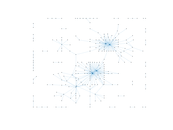Degree distribution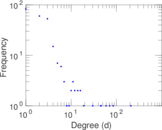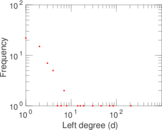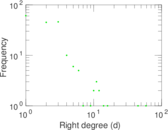Cumulative degree distribution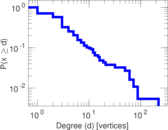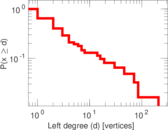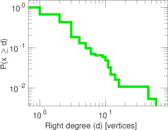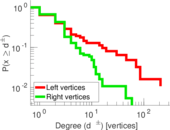Lorenz curve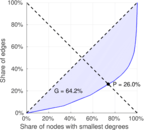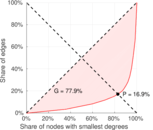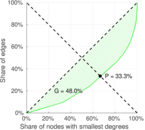Spectral distribution of the adjacency matrix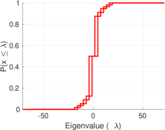Spectral distribution of the normalized adjacency matrix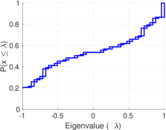Spectral distribution of the Laplacian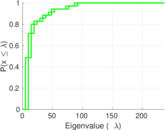Spectral graph drawing based on the adjacency matrix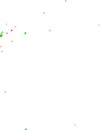Spectral graph drawing based on the Laplacian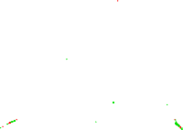Spectral graph drawing based on the normalized adjacency matrix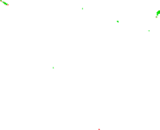Degree assortativity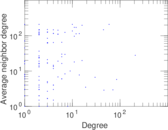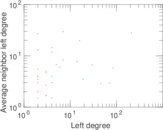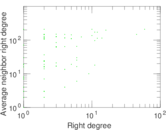Zipf plot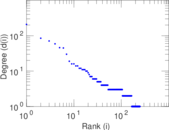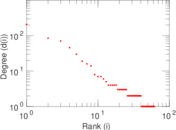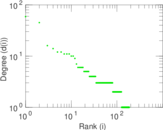Hop distribution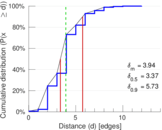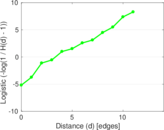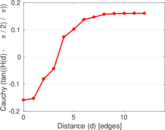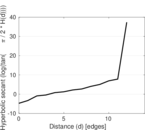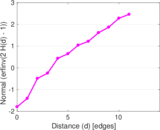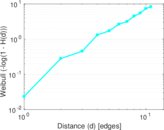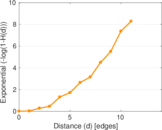Double Laplacian graph drawingDelaunay graph drawing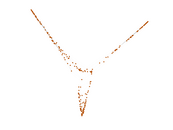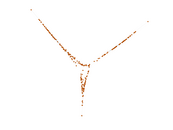Edge weight/multiplicity distribution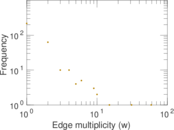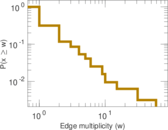Temporal distribution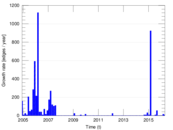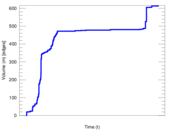Temporal hop distribution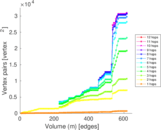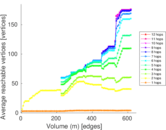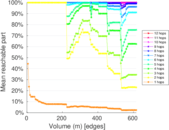Diameter/density evolution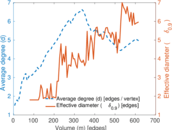Matrix decompositions plots